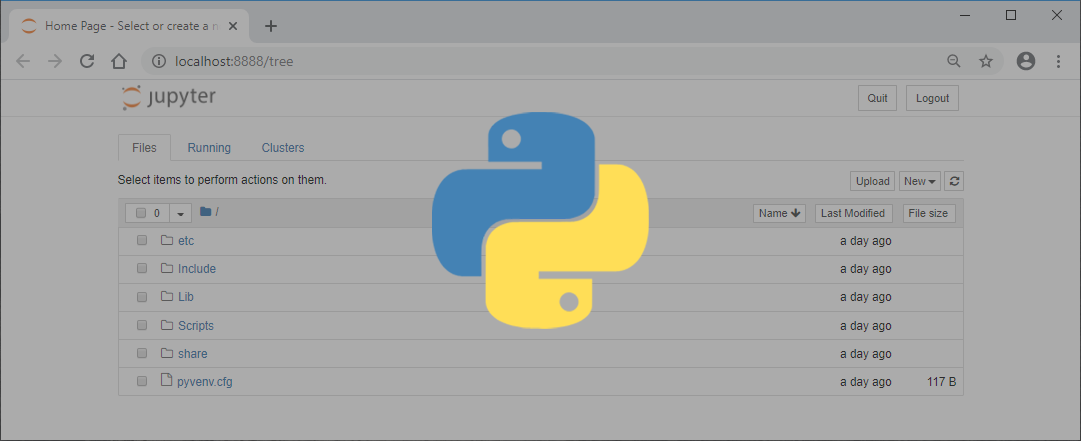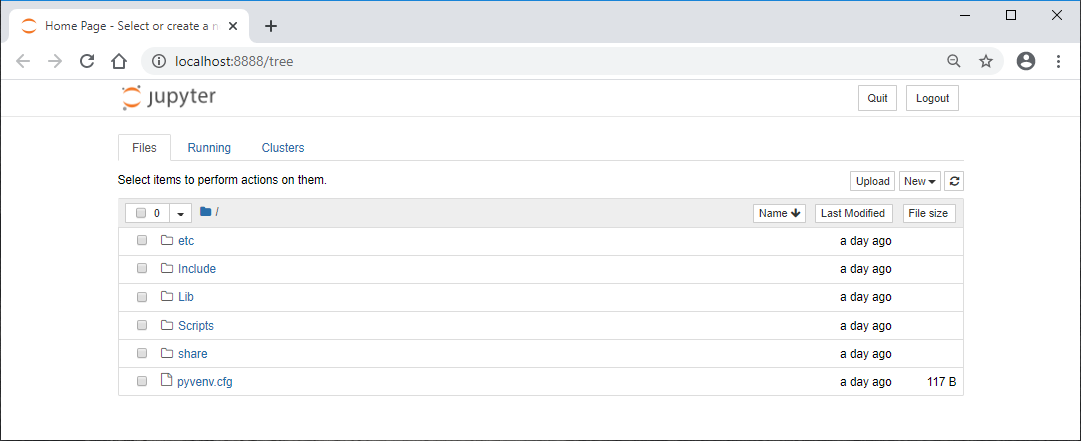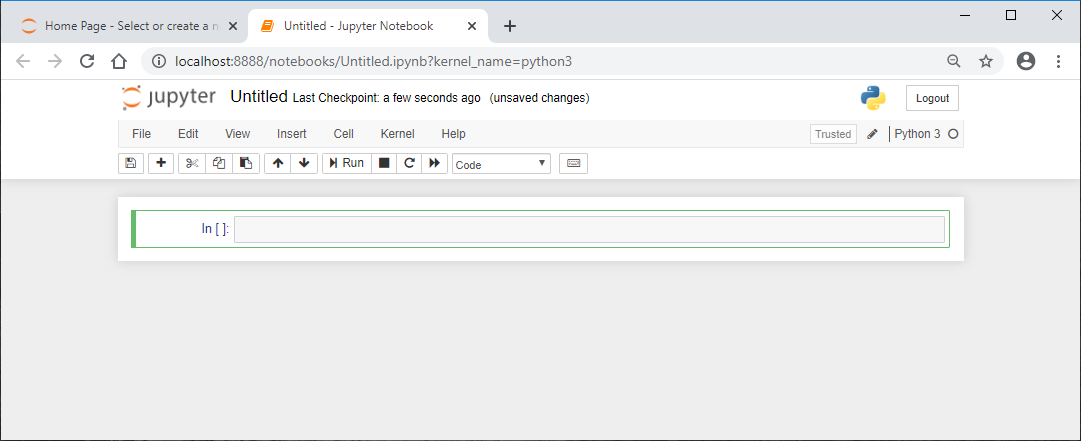# 25+ Helpful Python Commands in Jupyter Notebook

May 27, 2020, 11:09 a.m.

Beginners Python · 5 min readJupyter Notebook is an extremely helpful open-source web app used for all types of data transformation, modeling, and visualization. Below is a quick refresher on installing Jupyter Notebook and using Python commands to handle data sets within the app. Let's jump right in!

Note: For this tutorial, you will need to have Python programming language already installed on your computer.

Create and activate a virtual environment

macOS Terminal

``````User-Macbook:~ user\$ cd desktop

User-Macbook:desktop user\$ python3 -m venv jup

User-Macbook:desktop user\$ cd jup

User-Macbook:jup user\$ source bin/activate``````

Windows Command Prompt

``````C:\Users\Owner> cd desktop

C:\Users\Owner\desktop> py -m venv jup

C:\Users\Owner\desktop> cd jup

C:\Users\Owner\desktop\jup> Scripts\activate``````

First, type `python3 -m venv env` for Mac or `py -m venv env` for Windows to create a virtual environment named `jup`Then activate the virtual environment so you can properly install JupyterLab in the next step.

Install JupyterLab

macOS Terminal

``(jup)User-Macbook:jup user\$ pip install jupyterlab``

Windows Command Prompt

``(jup)C:\Users\Owner\desktop\jup> pip install jupyterlab``

Install JupyterLab, the interactive development environment for notebooks, with the command `pip install juypterlab`. This may take a couple of minutes.

Open JupyterLab in the browser

macOS Terminal

``(jup)User-Macbook:jup user\$ jupyter notebook``

Windows Command Prompt

``(jup)C:\Users\Owner\desktop\jup> jupyter notebook``

Once the installation is complete, open JupyterLab in your browser with the simple command `juypter notebook`.Your virtual environment folders will then appear in the browser window.

Create a new notebook

Click on the "New" button on the right side of the screen then select, "Python3". A notebook will then be created in a new tab.Install numpy pandas nltk

``````import sys
!{sys.executable} -m pip install numpy pandas nltk``````

Type in the command `pip install numpy pandas nltk` in the first cell. Click Shift + Enter to run the cell's code. An asterisk will then appear in the brackets indicating it is running the code.

When finished, a new cell will appear below. You are now ready to use Python commands to load-in and clean your data.

``````import pandas as pd

``````import pandas as pd

``````import pandas as pd

In a new cell `import pandas as pd`. Then set a variable, in this case `df` for dataframe, as `pd.read_doctype(r'full_path_to_file')`. Run the cell.

Working with columns

display column names

``df.columns``

Lists the names of all of the columns in the data frame.

display specific columns

``````df['column_name']

df['column_name1', 'column_name2', 'column_name3']``````

Display one specific column or multiple columns by calling on their names.

sort alphabetically within columns

``````df.sort_values('column_name')

df.sort_values('column_name', ascending=False)``````

Display the data frame alphabetically by the column specified. Add `ascending=False` to sort by descending alphabetical order.

drop/delete columns

``df = df.drop(columns = ['column_name']))``

Drops the column specified from the data set.

create a new column with addition

``df['new_column'] = df['column_name1'] + df['column_name2']``

Creates a new column equal to the sum of column 1 plus column 2 data.

Working with rows

display first rows

``````df.head()

``````

Displays the first 5 rows of the data frame. Add a number in the parentheses and that number of rows will display.

display last rows

``````df.tail()

df.tail(10)
``````

Displays the last 5 rows of the data frame. Add a number in the parentheses and that number of rows will display.

display a set of rows

``````df.iloc[0:5]
``````

Displays row 0-4 of the data frame. Note, the data frame starts counting rows/columns at 0 instead of 1.

Working with the columns and rows

locate a specific value by row and column

``df.iloc[2,1]``

Displays a specific value in a designated location. The example above locates the value in row 2, column 1.

locate specific rows by column

``df.loc[df['column_name'] == 'column_value']``

Displays all of the rows that meet the specified column value.

locate specific rows that adhere to all of the column values

``df.loc[(df['column_1'] == 'column_value') & df.loc(df['column_2'] == 'column_value')]``

Displays all rows with the specific column value of column 1 and the specific column value of column 2.

locate specific rows that adhere to one of the column values

``df.loc[(df['column_1'] == 'column_value') | df.loc(df['column_2'] == 'column_value')]``

Displays all rows with the specific column value of column 1 or the specific column value of column 2.

locate specific rows in a column that contain a certain word

``df.loc[df['column_1'].str.contains('word')]``

Lists all of the rows in column 1 that contain the word specified.

drop specific rows in a column that contain a certain word

``df.loc[~df['column_1'].str.contains('word')]``

Drop all of the rows in column 1 that contain the word specified.

Changing data types

view data types

``df.dtypes``

Outputs the data type (i.e. object, int32, datetime64...) of each column.

change data to integer

``df.column_name.astype(int)``

Changes the data type of the column specified from a string to an integer.

change data to datetime field

``pd.to_datatime(df.column_name)``

Changes the data type of the column specified from a string to a date/time integer.

Working with the data set as a whole

reset the index

``df.reset_index(drop=True, inplace=True)``

Resets the index of the data frame. This works well if you delete or change the ordering of rows.

list duplicates

``df.drop_duplicates()``

Identifies duplicates in the data set.

drop duplicates

``df.drop_duplicates()``

Drops any duplicate rows from the data set.

combine datasets

``data = pd.concat([df1, df2, df3])``

Combines all of the data from each data frame into a new data set.

Saving updated data frames as files

JSON file

``df.to_json('name_of_new_file.json')``

Save the existing data frame as a new CSV file.

CSV file

``df.to_csv('name_of_new_file.csv')``

Save the existing data frame as a new CSV file.

excel file w/o the index

``df.to_excel('name_of_new_file.xlsx', index=False)``

Save the existing data frame as a new excel file without the index numbers. Note, `index=False` can be added to the other.

0
##### Subscribe now

Subscribe to stay current on our latest articles and promos